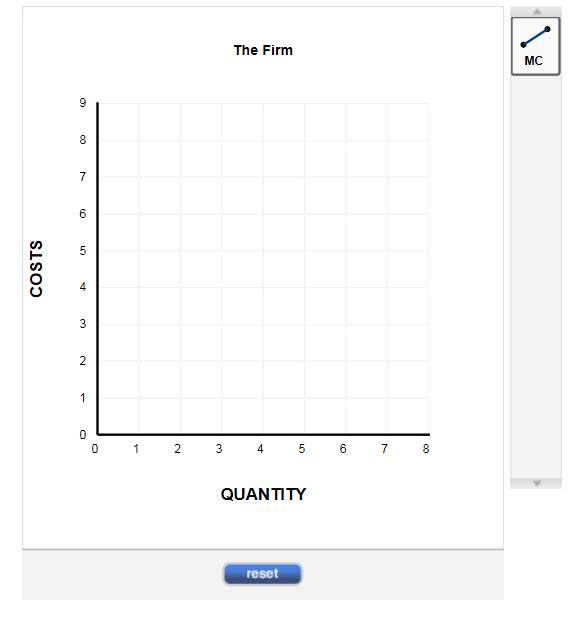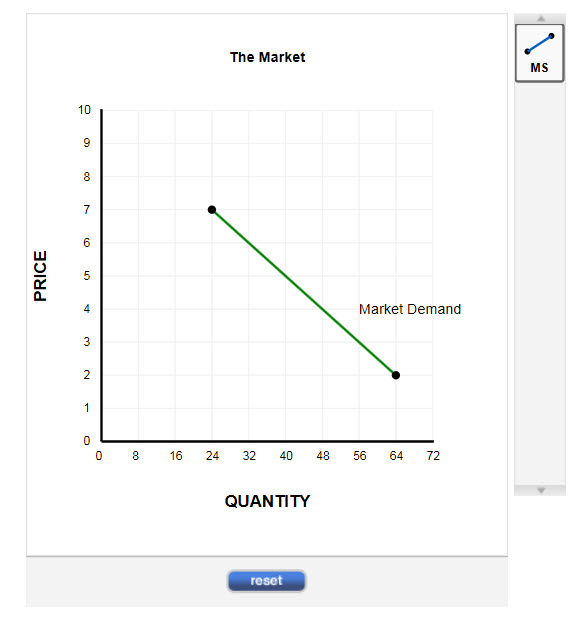Chat Now

# Suppose the following data summarize the costs of a perfectly competitive

### Suppose the following data summarize the costs of a perfectly competitive

Suppose the following data summarize the costs of a perfectly competitive firm:

 Quantity 0 1 2 3 4 5 6 7 8 Total cost \$100 \$101 \$103 \$106 \$110 \$115 \$121 \$128 \$136

(a) Draw the firmAc€?cs marginal cost curve.

Instructions: Use the tool provided to draw the marginal cost curve (MC). This line should only contain the two endpoints.(b) Draw the market supply curve on the graph, assuming 8 firms identical to the one described in the table above.

Instructions: Use the line tool provided to draw the market supply curve (MS). This line should only contain the two endpoints.(c) What is the equilibrium price in this market?

\$ _________________

abhinav behal 26-May-2020Get solution